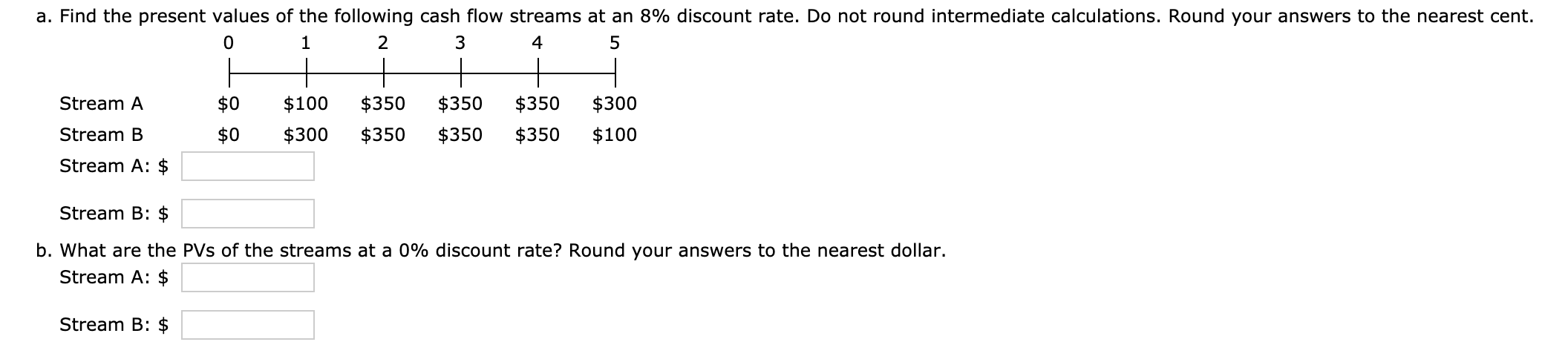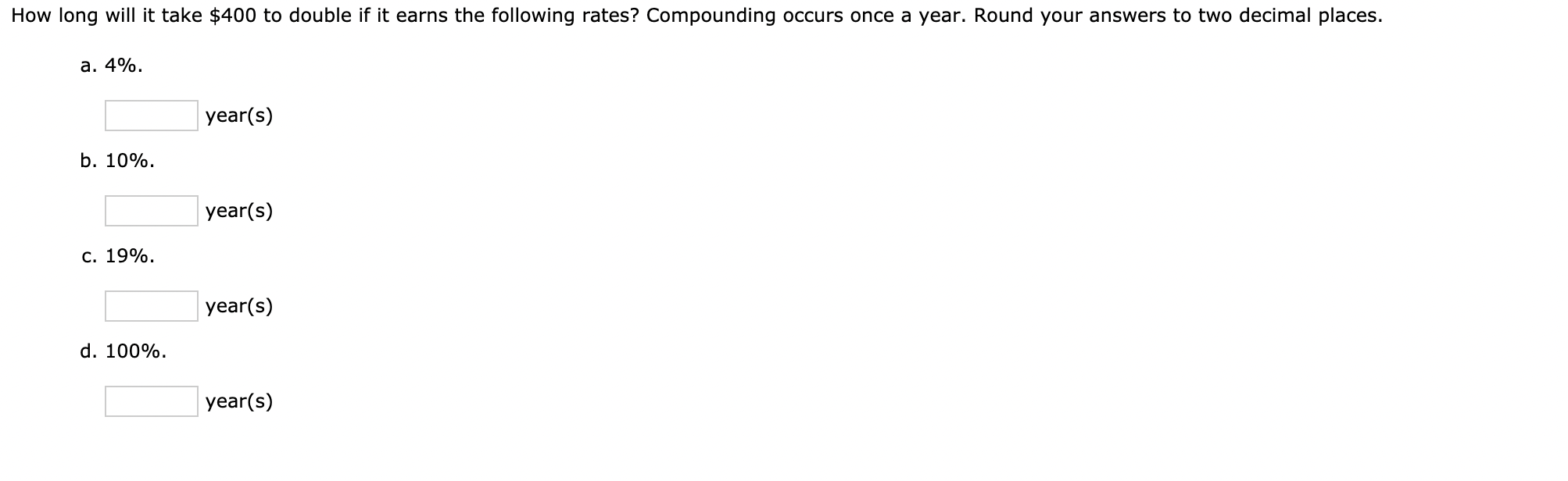# Question a. Find the present values of the following cash flow streams at an 8% discount rate. Do not round intermediate calculations. Round your answers to the nearest cent. 0 1 2 3 4 5 Stream A $0$0 $100$300 $350$350 $350$350 $350$350 $300$100 Stream B Stream A: $Stream B:$ b. What are the PVs of the streams at a 0% discount rate? Round your answers to the nearest dollar. Stream A: $Stream B:$ How long will it take $400 to double if it earns the following rates? Compounding occurs once a year. Round your answers to two decimal places. a. 4%. year(s) b. 10%. year(s) c. 19%. year(s) d. 100%. year(s)53FOFC The Asker · FinanceTranscribed Image Text: a. Find the present values of the following cash flow streams at an 8% discount rate. Do not round intermediate calculations. Round your answers to the nearest cent. 0 1 2 3 4 5 Stream A$0 $0$100 $300$350 $350$350 $350$350 $350$300 $100 Stream B Stream A:$ Stream B: $b. What are the PVs of the streams at a 0% discount rate? Round your answers to the nearest dollar. Stream A:$ Stream B: $How long will it take$400 to double if it earns the following rates? Compounding occurs once a year. Round your answers to two decimal places. a. 4%. year(s) b. 10%. year(s) c. 19%. year(s) d. 100%. year(s)
More
Transcribed Image Text: a. Find the present values of the following cash flow streams at an 8% discount rate. Do not round intermediate calculations. Round your answers to the nearest cent. 0 1 2 3 4 5 Stream A $0$0 $100$300 $350$350 $350$350 $350$350 $300$100 Stream B Stream A: $Stream B:$ b. What are the PVs of the streams at a 0% discount rate? Round your answers to the nearest dollar. Stream A: $Stream B:$ How long will it take $400 to double if it earns the following rates? Compounding occurs once a year. Round your answers to two decimal places. a. 4%. year(s) b. 10%. year(s) c. 19%. year(s) d. 100%. year(s) Community AnswerYHHDBT Answer:a. Calculation of the PV of the streams at an 8% discount rate:Formula:Present value = Future value xx1//(1+" rate ")^(^^)nWhere n is year of cash flowStream AStream BSo, the PV of the stream at 8% is$1,131.94So, the PV of the stream at 8% is $1,181.01b. Calculation of the PV of the streams at an 0% discount rate:Stream AStream BSo, the PV of the stream at 0% is$1450So, the PV of the stream at 0% is $1450 Answer:Calculation of the time it will$400 to double:Syntax we will use here =quad= NPER(rate,-pmt, pv,-fv, type ... See the full answer